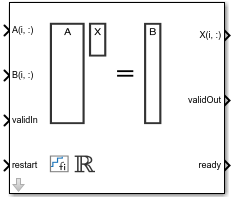Real Burst Matrix Solve Using QR Decomposition

Compute the value of x in the equation Ax = B for real-valued matrices using QR decomposition

• Library:
• Fixed-Point Designer HDL Support / Matrices and Linear Algebra / Linear System Solvers

•Description

The Real Burst Matrix Solve Using QR Decomposition block solves the system of linear equations Ax = B using QR decomposition, where A and B are real-valued matrices. To compute x = A-1, set B to be the identity matrix.

Ports

Input

expand all

Rows of real matrix A, specified as a vector. A is an m-by-n matrix where m ≥ 2 and mn. If B is single or double, A must be the same data type as B. If A is a fixed-point data type, A must be signed, use binary-point scaling, and have the same word length as B. Slope-bias representation is not supported for fixed-point data types.

Data Types: single | double | fixed point

Rows of real matrix B, specified as a vector. B is an m-by-p matrix where m ≥ 2. If A is single or double, B must be the same data type as A. If B is a fixed-point data type, B must be signed, use binary-point scaling, and have the same word length as A. Slope-bias representation is not supported for fixed-point data types.

Data Types: single | double | fixed point

Whether inputs are valid, specified as a Boolean scalar. This control signal indicates when the data from the A(i,:) and B(i,:) input ports are valid. When this value is 1 (true) and the value at ready is 1 (true), the block captures the values on the A(i,:) and B(i,:) input ports. When this value is 0 (false), the block ignores the input samples.

After sending a true validIn signal, there may be some delay before ready is set to false. To ensure all data is processed, you must wait until ready is set to false before sending another true validIn signal.

Data Types: Boolean

Whether to clear internal states, specified as a Boolean scalar. When this value is 1 (true), the block stops the current calculation and clears all internal states. When this value is 0 (false) and the validIn value is 1 (true), the block begins a new subframe.

Data Types: Boolean

Output

expand all

Rows of the matrix X, returned as a scalar or vector.

Data Types: single | double | fixed point

Whether the output data is valid, returned as a Boolean scalar. This control signal indicates when the data at the output port X(i,:) is valid. When this value is 1 (true), the block has successfully computed a row of matrix X. When this value is 0 (false), the output data is not valid.

Data Types: Boolean

Whether the block is ready, returned as a Boolean scalar. This control signal indicates when the block is ready for new input data. When this value is 1 (true) and the validIn value is 1 (true), the block accepts input data in the next time step. When this value is 0 (false), the block ignores input data in the next time step.

After sending a true validIn signal, there may be some delay before ready is set to false. To ensure all data is processed, you must wait until ready is set to false before sending another true validIn signal.

Data Types: Boolean

Parameters

expand all

Number of rows in input matrices A and B, specified as a positive integer-valued scalar.

Programmatic Use

 Block Parameter: m Type: character vector Values: positive integer-valued scalar Default: 4

Number of columns in input matrix A, specified as a positive integer-valued scalar.

Programmatic Use

 Block Parameter: n Type: character vector Values: positive integer-valued scalar Default: 4

Number of columns in input matrix B, specified as a positive integer-valued scalar.

Programmatic Use

 Block Parameter: p Type: character vector Values: positive integer-valued scalar Default: 1

Data type of the output matrix X, specified as fixdt(1,18,14), double, single, fixdt(1,16,0), or as a user-specified data type expression. The type can be specified directly, or expressed as a data type object such as Simulink.NumericType.

Programmatic Use

 Block Parameter: OutputType Type: character vector Values: 'fixdt(1,18,14)' | 'double' | 'single' | 'fixdt(1,16,0)' | '' Default: 'fixdt(1,18,14)'

Tips

Use fixed.getMatrixSolveModel(A,B) to generate a template model containing a Real Burst Matrix Solve Using QR Decomposition block for real-valued input matrices A and B.

Extended Capabilities

Introduced in R2019b

Fixed-Point Designer DocumentationGet trial now